# Crank mechanism kinetics - Crank effort and balancing

This tutorial concerning slider and crank mechanisms covers two aspects directly related to the operation of actual machines.

We look firstly at output characteristics of a crankshaft and flywheel under torque load conditions using crank effort diagrams.  We then consider the balancing of inertial forces and moments generated by the mechanism.

#### Crank effort

Consider the crank mechanism as an engine operating at constant angular velocity ω against a constant torque load.  We use the mechanism with parameters from previous tutorials shown below but with the driving force increased by a factor of 10 to the more practical value of 10 kN.  The horizontal driving force FP at the slider is constant and "double acting", i.e. the direction of the force reverses at θ = 180°.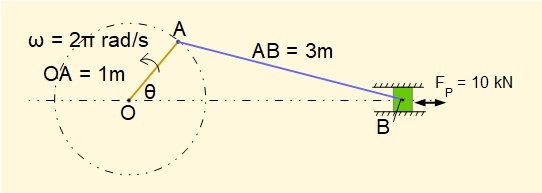The plot below (called a crank effort diagram) shows the fluctuating torque generated by the engine at the crankshaft (called the engine torque) over one rotational cycle, derived from first principles shown in a previous tutorial.  For simplicity we ignore the effects of inertia forces and moments.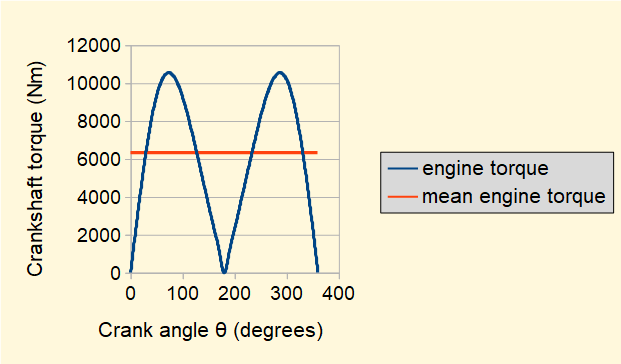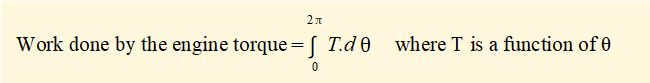Hence the area under the engine torque plot is the work done in one crank cycle which we calculate by numerical integration to be 39900 Nm.  Thus at constant angular velocity ω = 2π rad/s the power output = 39.9 kW.

We represent the same amount of work being done by a constant torque Tav called the mean engine torque  The calculated value = 6350 Nm is shown on the plot.

Tav is also the constant load torque on the engine running at nominally constant angular velocity ω.  Given the highly fluctuating engine torque how can constant (or near constant) angular velocity be maintained?  The answer is by the rotational inertia of a flywheel and other rotating masses with rotational inertia in the drive chain.

Referring to the crank effort diagram above, where engine torque is greater than mean engine torque the engine provides energy to the flywheel which increases ω.  Conversely, where engine torque is less than mean engine torque the flywheel provides energy to the engine which causes ω.to reduce  Thus ω fluctuates between upper and lower values over one cycle.

We now estimate the variation in ω occurring with flywheel having mass moment of inertia IFY.

Let the highest speed = ωmax and lowest speed = ωmin

Consider the diagram below.  From the crank effort diagram we identify which segment of the cycle has the maximum fluctuation in energy EFL relative to the mean engine torque.  From numerical integration we establish that segment B has the largest fluctuation EFL = 5910 Nm .  Thus ωmax  occurs at the transition point between segments A and B and ωmin at the transition between segments B and C.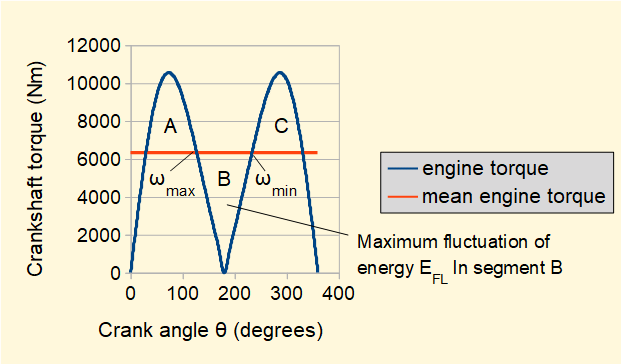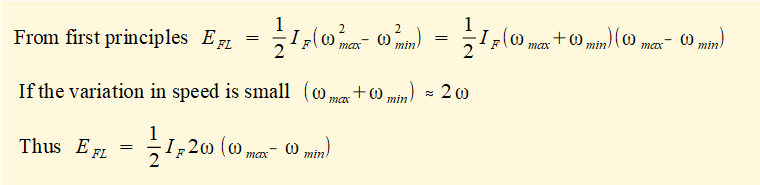For this example a spoked annular ring flywheel shown above which is twice the diameter of the crank arm and has mass moment of inertia IFY = 6000 kgm2 is attached to the crankshaft.  Using the above expression gives the following result  for EFL = 5910 Nm

(ωmax = ωmin)  = 0.157 rad/s = 1.50 revs/min (equivalent to ω = ± 0.75 revs/min)

Note the following terms.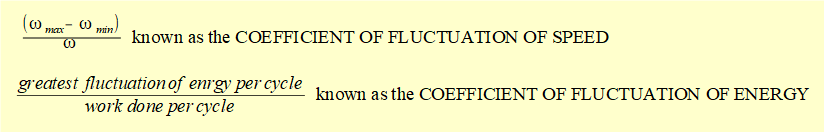In example above the coefficient of fluctuation of speed = 0.025 or 2.5% and the coefficient of fluctuation of energy = 0.148 or 14.8%.  These are typical values for double acting, single cylinder steam engines.

However a high degree of control over output speed comes at a cost as the flywheel has a large mass (c.1500 kg) and the high mass moment of inertia makes the engine difficult to start.

To achieve the same output with a smaller flywheel we now consider three identical engines with the crank arms 120° apart on the same crankshaft and the driving force FP at each engine = 3.33 kN.  The crank effort diagram below shows the effect.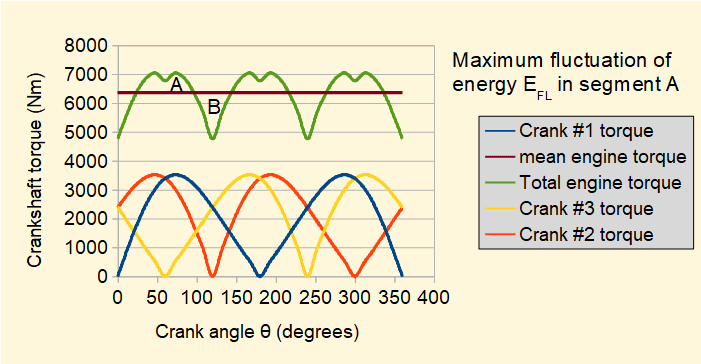The mean engine torque is unchanged but the maximum fluctuation of energy EFL by numerical integration = 612 Nm giving a coefficient of fluctuation of energy = 1.5% as opposed to 14.8% for a single crank.

The same coefficient of fluctuation of speed = 2.5% is achieved with a flywheel of identical annular cross section but approximately half the diameter and mass, the consequence of a ten fold decrease in the mass moment of inertia.  Note that it is possible to start the engine at almost full load torque.

The example above is relatively simple based on an idealised "engine".  Crank effort diagrams for multi-cylinder, two and four stroke  internal combustion engines are significantly more complex.

#### Balancing

In a previous tutorial we examined inertia forces and moments generated in a slider and crank mechanism and estimated their effect on crankshaft torque and reaction forces at bearings.  Fluctuating forces associated with inertial effects can generate vibrations which are transmitted to the machine supports.  Measures to neutralise these effects are known as balancing.

Firstly,  a brief summary of the basic principles of balancing.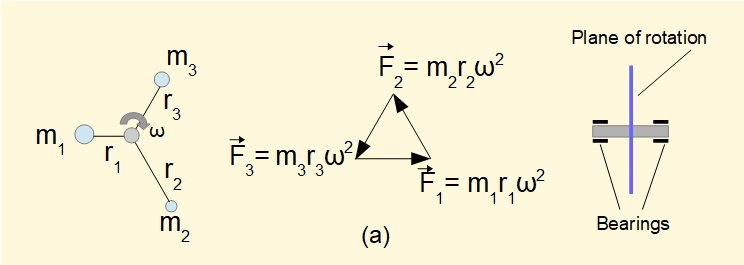In diagram (a) above masses mi are attached by massless rods of radius ri to a shaft rotating with angular speed ω .  The centripetal acceleration riω2 of each mass is associated with a tension force along each rod = miriω2 and corresponding reaction forces on the rotating masses and shaft bearings*.  In this case the sum of these force vectors is zero thus there is no resultant reaction force at the shaft bearings arising from rotation of the masses.  In this state we say that the rotating shaft is in static balance.  The general requirement for static balance of rotating masses is Σmiri = 0 (considering r as a vector).   The term static is appropriate since the rotor is in static equilibrium at all angular positions of the shaft.

This condition can also be expressed by saying that the vector sum of the centrifugal inertial forces* for the masses is zero.

* The tension force along the rod accounts for the centripetal acceleration of the rotating mass directed towards the centre of rotation  The diagrams show the reaction forces of this tension acting on the rotating masses.  Centrifugal inertial forces acting on the masses, directed away from the centre of rotation, are "pseudo forces" which allow analysis of accelerating masses using the condition of static equilibrium. ( D'Alembert's principle - see previous tutorial).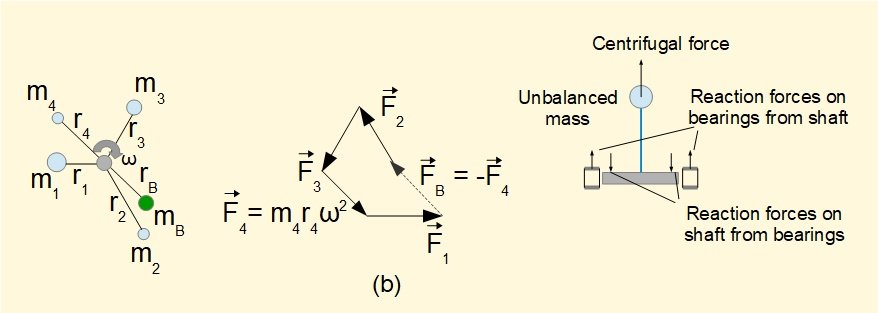In diagram (b) an additional mass m4 is attached to the rotor. Now the vector sum of tension forces in the rods is not zero.  The rotor is in a state of static unbalance and a net force arises on the rotor shaft bearings.   In this example the unbalanced force = F4.  A balancing mass mB can be added to restore static balance such that mBrBω2  = -m4r4ω2  .

The diagram below illustrates another requirement known as dynamic balancing.In the diagram above the rotor with attached masses of equal mass m at radius r meets the condition for static balance as Σmiri = 0 for all angles of rotation.

However, the lower diagrams show a state of dynamic unbalance where centrifugal inertial forces mω2r exert a moment on the shaft with  resultant reaction forces on the bearings.  The directions of the bearing reaction forces rotate with the shaft;  directions of forces at rotation angles of 0° and 180° are shown on the diagram. The location of the rotating masses along the axis of the shaft determines the magnitude of the bearing forces, the further apart the rotational planes the larger the forces.  In the limiting case when the rotating planes converge, reaction forces are zero.

Dynamic balancing involves placing additional mass on the rotor at positions such that the net moment on the shaft is zero. It is particularly important in the design of multi-cylinder engines.  In this tutorial we examine only static unbalance generated in a single crank arrangement.

#### Balancing a single slider and crank mechanism

In a previous tutorial we identified inertial forces generated by the three moving elements of a slider and crank mechanism:

• Rotational inertial force generated by the crank arm
• Reciprocating (translational) inertial force generated by the slider
• Inertial force and moment generated by the connecting rod

We examine balancing these inertial forces and moments from each element in turn using the example mechanism below.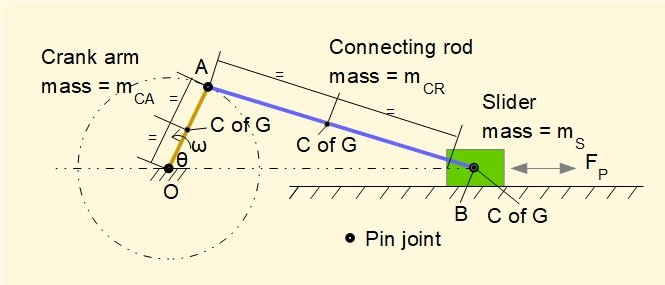Individual elements are as follows.  The C of G is located at the mid-point of each element.

• Mass of slider mS = 10 kg
• Mass of connecting rod mCR = 5 kg        Length of connecting rod R = 3 m
• Mass of crank arm  mCA = 2 kg                Length of crank arm  L = 1 m

#### Crank arm

Referring to the diagram below the inertial centrifugal force FIca experienced by the crank arm rotating at constant angular velocity ω produces a rotating unbalanced force acting through point O.  In this example the C of G of the crank arm is at position  R/2.  Thus FICA = mCAω2(R/2) = 2 x (2π2) x 0.5 =  19.7 N

We can balance this force by placing a rotating counterbalance mass mB at radius RB diametrically opposed to the crank arm such that mB x RB = mCA x (R/2) giving for this example  mB = 1/RB kg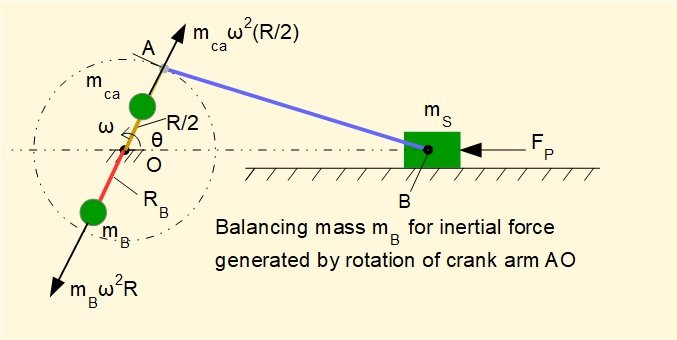#### Slider

The acceleration aB of the reciprocating slider of mass mS produced by applied force FP generates an inertial force FIS such that FIS = - (mS x aB)

In a previous tutorial we derived the following expression for the acceleration of the slider, aB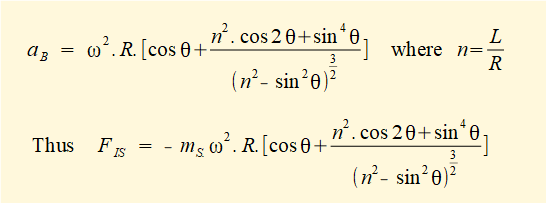In this previous tutorial we derived the above expression for aB by successive differentiation of the linear displacement of point B as a function of crank angle θ.  When considering balancing it is convenient to express the second term in brackets as follows*.

* This approximation is obtained by applying the binomial theorem to the expression for displacement of point B.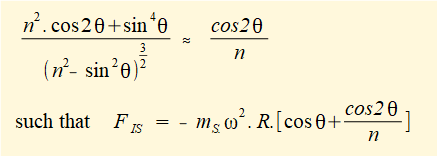We now consider the inertial force generated by the slider as two separate parts: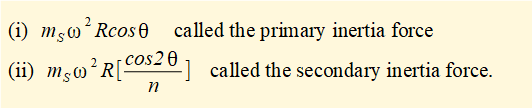Primary inertia force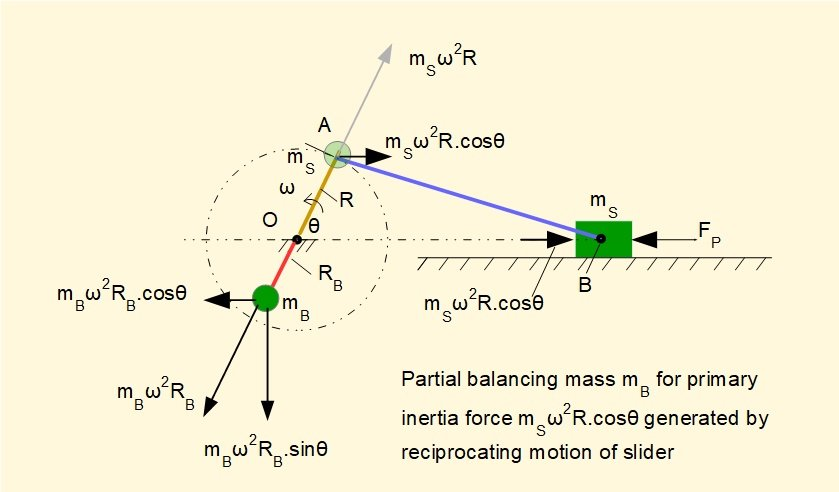The diagram above shows the primary inertia force mSω2Rcosθ generated by the acceleration of mass mS at crank angle θ.  This force varies in magnitude and direction through the complete crank cycle as a function of cosθ.  In this example the maximum value of this primary force component (at θ = 0° and 180° ) = 10 x (2π)2 x 1 = 395 N

This force can be seen as equivalent to the horizontal component of the centrifugal force generated by an imaginary mass mS rotating about point O at radius R which can be balanced by the horizontal component of the centrifugal force generated by a diametrically opposed counterbalance mass mB at radius RB such that mB x RB = mS x R.

However, the vertical component of the centrifugal force generated by the counterbalance mass mB creates an unbalanced force = mBω2RBsinθ.  In practical terms it is a question whether suppression of an oscillating force in in the horizontal plane outweighs the adverse effect of creating an oscillating force in the vertical plane.

Secondary inertia forcewhich is a function of cos2θ and can be interpreted as the horizontal component of the centrifugal force generated by slider mass mS rotating on a secondary imaginary crank arm with angular velocity 2ω such that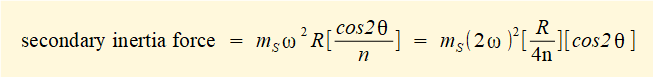Thus the radius of this secondary crank = R/4n.  This force can be balanced by counterbalance mass mB on a diametrically opposed secondary crank arm at radius RB such that mS x (R/4n) = mB x RB as shown in the diagram below.  In common with the counterbalance for the primary inertia force this method of balancing introduces an unbalanced vertical force = mB(2ω)2RBsinθ.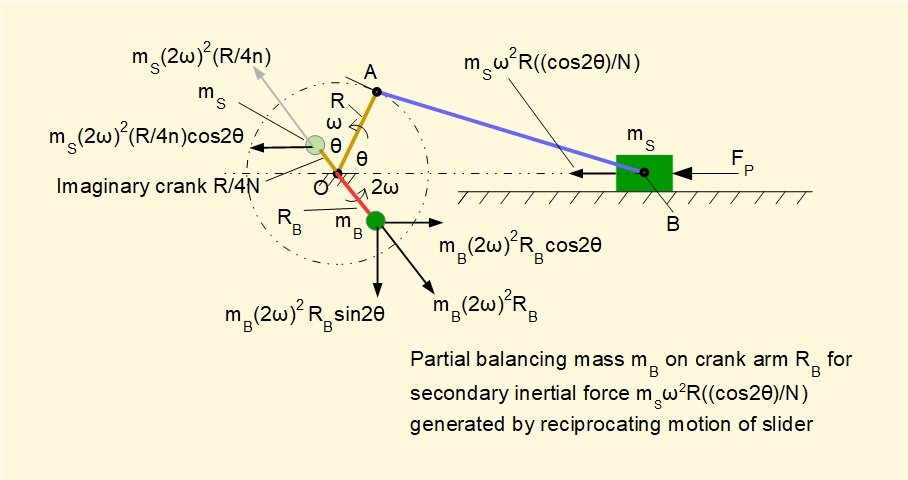The difficulty of constructing a practical arrangement for a secondary crank is such that balancing the secondary inertia force is rarely carried out.

Connecting rod

In a previous tutorial we examined the inertial force and torque (or moment) generated by the connecting rod shown in the diagram below for a specific crank angle θ.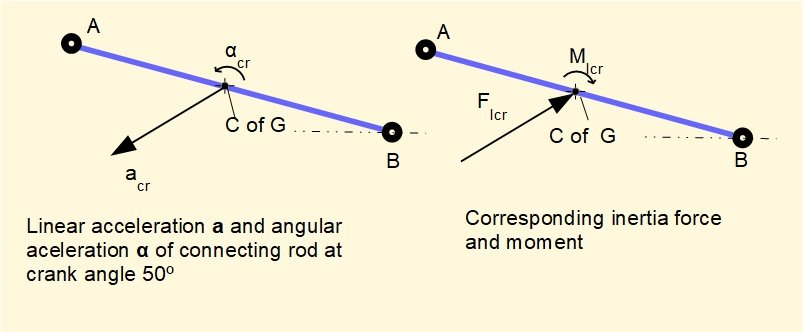We now convert this inertia force and moment io an equivalent dynamic system representing the total mass of the connecting rod m as two point masses m1 and m2 conveniently located at points B and A connected by a massless link as shown below.  We will then add the inertial forces generated by m1 and m2 respectively to the inertial forces generated by the slider and crank arm as above.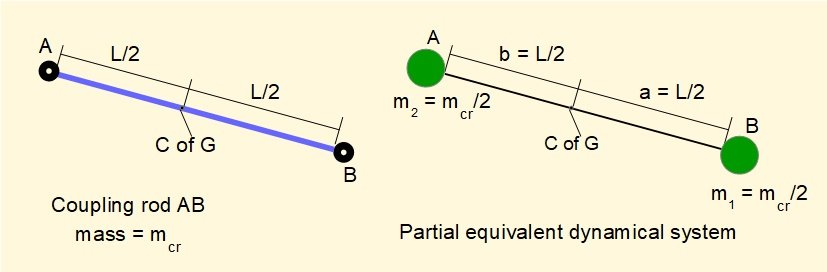The general criteria for an equivalent dynamic system with reference to the parameters of right hand diagram above are:

1. m = m1 + m2
2. m1 x a = m2 x b
3. m1  x a2 + m2 x b2 = m x.k2    where k is the radius of gyration of the component rotating about an axis through its centre of gravity

For the case above where m1 = m2 = m/2 and  a = b = L/2 it is clear that No.1 and No.2 are satisfied.

For No.3 to be satisfied:

m1 x L2/4 + m2 x L2/4 = L2/4(m1 + m2) = m (L2/4)

For a slender rod rotating on an axis through its C of G,  k = L//√12    giving  m x k2 = m(L2/12)

Clearly No.3 is not satisfied*1 with this arrangement of masses*2.   However, partial dynamic equivalence does provide an approximate measure of the unbalance generated by the inertia of the coupling rod.

*1  Complete dynamic equivalence can be obtained by applying a "correction couple" = m(a.b = k2).α where α is the angular acceleration of the connecting rod.  Since α varies continuously the correction has limited value for the purpose of determining any counterbalancing mass.

*2  An equivalent dynamic system satisfying all three conditions with one mass located at point A or point B and the other mass located elsewhere on the rod axis does exist but this arrangement of masses does not suit our purpose.

Inertia forces generated by masses m1 and m2 of the equivalent dynamic system and the corresponding counterbalancing masses are shown in the diagrams below.  The counterbalance mass for m2 can be added to the counterbalance mass for the rotational inertia force generated by the crank arm.  The counterbalance mass for m1 can be added to the counterbalance mass for the reciprocating primary inertia force generated by slider mass ms.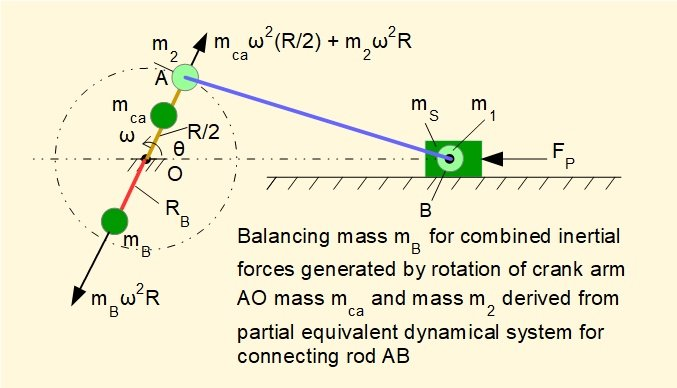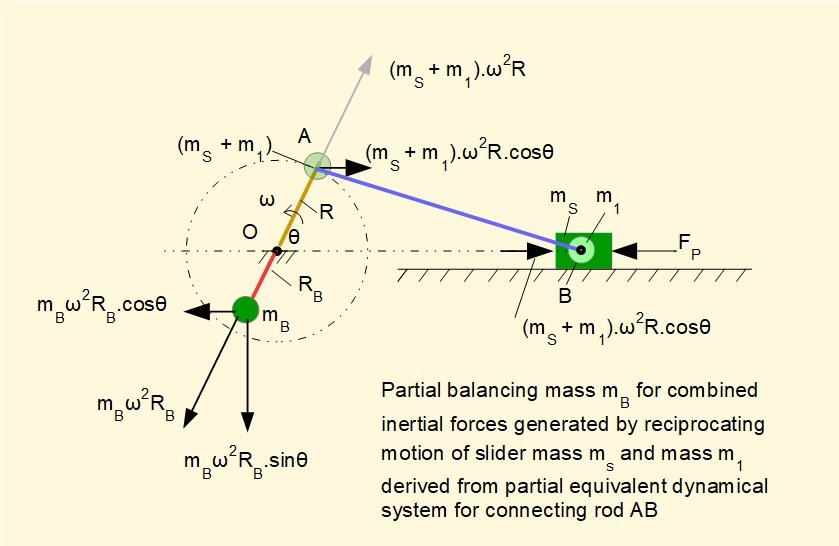I welcome feedback at:

### Tutorials - mechanical vibrations

###### Forced vibrations with damping

Alistair's tutorials 2020